FanDuel 2019 Western Conference Odds
+340
3.4 to 1Avalanche
18.8% implied probability

+340
3.4 to 1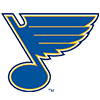Blues
18.8% implied probability

+600
6 to 1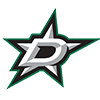Stars
11.8% implied probability

+750
7.5 to 1Golden Knights
9.7% implied probability

+1100
11 to 1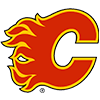Flames
6.9% implied probability

+1100
11 to 1Predators
6.9% implied probability

+1400
14 to 1Coyotes
5.5% implied probability

+1500
15 to 1Canucks
5.2% implied probability

+1700
17 to 1Oilers
4.6% implied probability

+1700
17 to 1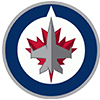Jets
4.6% implied probability

+2900
29 to 1Wild
2.8% implied probability

+4600
46 to 1Sharks
1.8% implied probability

+5000
50 to 1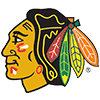Blackhawks
1.6% implied probability

+12000
120 to 1Ducks
0.7% implied probability

+14000
140 to 1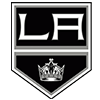Kings
0.6% implied probability